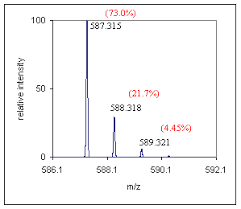## How to Calculate and Solve for the Quantity of Charge, Electrochemical Equivalence of a Substance and Mass of an Element in Electrolysis | Nickzom CalculatorThe image above represents the mass of an element.

To compute for the mass of an element, two essential parameters are needed and these parameters are Electrochemical Equivalence of the Substance (Z) and quantity of charge (Q).

The formula for calculating mass of an element:

M = ZQ

Where;
M = Mass of the element
Z = Electrochemical Equivalence of the Substance
Q = Quantity of Charge

Let’s solve an example;
Find the mass of an element when the Quantity of charge is 28 and Electrochemical Equivalence of the Substance is 32.

This implies that;
Z = Electrochemical Equivalence of the Substance = 32
Q = Quantity of Charge = 28

M = ZQ
M = 32 x 28
M = 896

Therefore, the mass of an element is 896 kg.

Calculating the Electrochemical Equivalence of the Substance when the Mass of an Element and Quantity of Charge is Given.

Z = M / Q

Where;
Z = Electrochemical Equivalence of the Substance
M = Mass of the element
Q = Quantity of Charge

Let’s solve an example;
Find the Electrochemical Equivalence of the Substance when the Quantity of charge is 12 and  mass of an element  is 120.

This implies that;
M = Mass of the element = 120
Q = Quantity of Charge = 12

Z = M / Q
Z = 120 / 12
Z = 10

Therefore, the Electrochemical Equivalence of the Substance is 10.